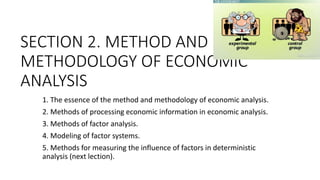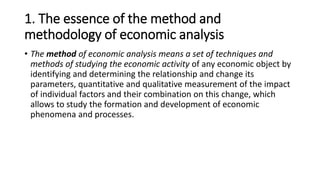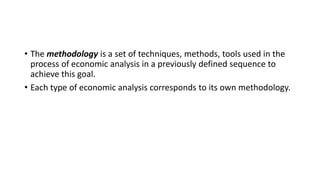Diese Präsentation wurde erfolgreich gemeldet.
Anzeige
Anzeige
Anzeige
Anzeige
Anzeige
Anzeige
Anzeige
Anzeige
Anzeige
Anzeige
Anzeige×

1 von 25 Anzeige

# lecture 2.pptx

https://econ.biem.sumdu.edu.ua/
1. The essence of the method and methodology of economic analysis.
2. Methods of processing economic information in economic analysis.
3. Methods of factor analysis.
4. Modeling of factor systems.
5. Methods for measuring the influence of factors in deterministic analysis (next lection).

https://econ.biem.sumdu.edu.ua/
1. The essence of the method and methodology of economic analysis.
2. Methods of processing economic information in economic analysis.
3. Methods of factor analysis.
4. Modeling of factor systems.
5. Methods for measuring the influence of factors in deterministic analysis (next lection).

Anzeige
Anzeige

### lecture 2.pptx

1. 1. SECTION 2. METHOD AND METHODOLOGY OF ECONOMIC ANALYSIS 1. The essence of the method and methodology of economic analysis. 2. Methods of processing economic information in economic analysis. 3. Methods of factor analysis. 4. Modeling of factor systems. 5. Methods for measuring the influence of factors in deterministic analysis (next lection).
2. 2. 1. The essence of the method and methodology of economic analysis • The method of economic analysis means a set of techniques and methods of studying the economic activity of any economic object by identifying and determining the relationship and change its parameters, quantitative and qualitative measurement of the impact of individual factors and their combination on this change, which allows to study the formation and development of economic phenomena and processes.
3. 3. • The methodology is a set of techniques, methods, tools used in the process of economic analysis in a previously defined sequence to achieve this goal. • Each type of economic analysis corresponds to its own methodology.
4. 4. • Under the general methodology is understood a system of research that is equally used in the study of different objects of economic analysis in different sectors of the economy. • Some methodologies specify the general in terms of industries, type of production or object of study.
5. 5. • Methods of economic analysis are divided into: • - logical (traditional); • - methods of factor analysis; • - methods of optimization of economic problems.
6. 6. 2. Methods of processing economic information in economic analysis Among the traditional methods of economic analysis can be identified methods that are most widely used for processing and studying information - it’s: • comparison, • balance, • grouping, • graphical techniques, • heuristic methods.
7. 7. • Comparison is a method by which the object (phenomenon) being studied is characterized by the ratio, measurement, comparison with other similar objects or phenomena. • Grouping is a method of analysis, which consists in the formation of an array of data analyzed, classification groups on the grounds that are important in terms of solving specific analytical problems.
8. 8. • The balance method has become widespread as a scientific method of accounting. • The use of the balance sheet method is based on the limitations, finiteness of the amount of material resources and rigid relationships between the individual elements of the population, which arise in this regard. • The balance of marketable products, different balances of individual deviations, the consistency of factors is studied.
9. 9. Consider the application of the balance sheet method for compiling a trade balance, the formula of which has the form • 𝑁поч + 𝑁над = 𝑁реаліз + 𝑁кінц, • Nпоч - balance of products at the beginning of the period; • Nнад - receipt of finished products from production; • Nреаліз - volume of sold products; • Nкінц - balance of products at the end of the period. Based on the formula of the commodity balance, the volume of sold products (Nреаліз) will be determined by the formula кінц над поч реаліз N N N N   
10. 10. • Graphic techniques are used to better perceive and understand economic phenomena and processes, as well as trends and patterns of change and development. Graphs (diagrams) are a visual representation of data with the help of geometric signs, figures and other graphical means, which conditionally express numerical indicators and the relationship between them. • Heuristic techniques include: reception of collective expert assessment, reception of analogy, reception of inversion, reception of brainstorming, morphological analysis, reception of control questions, reception of a collective notebook.
11. 11. 3. Methods of factor analysis. There are the following types of factor analysis: • deterministic (functional) and stochastic (correlation); • direct (deductive) and reverse (inductive); • single-stage and multi-stage; • static and dynamic; • retrospective and perspective (predictive).
12. 12. • Determined factor analysis is a technique for investigating the influence of factors whose relation to the resultant index is functional, that is, the resultant index can be represented as the product, fraction or algebraic sum of factors.
13. 13. • Stochastic analysis is a technique for investigating factors whose relation to a performance index, in contrast to a functional one, is incomplete, probabilistic (correlative).
14. 14. • Direct factor analysis involves deductive research, from general to individual. • Turning to the inverse factor analysis, we will study the study of cause and effect relationships by the method of logical induction - from individual factors to generalizations, from cause to effect in order to determine the sensitivity of changes in many performance indicators to changes in the studied factor.
15. 15. • Factor analysis can be one-tier and multi-tier. • One-tier factor analysis is used to investigate factors of only one level of subordination without detailing them into components. For example, y = a*b. • In multi-tier factor analysis, the factors a and b are disaggregated into constituent elements in order to study their nature.
16. 16. • It is necessary to distinguish between static and dynamic factor analysis. • Static factor analysis is used to examine the effect of factors on performance before the relevant date. • Dynamic factor analysis is a technique for investigating cause and effect relationships in dynamics.
17. 17. • Finally, factor analysis can be retrospective, exploring the causes of changes in economic performance in past periods, and prospective, exploring the behavior of factors and performance indicators for the future.
18. 18. • The main stages of factor analysis are follows: • 1. The choice of factors that influence the studied performance indicators; their classification and systematization to provide a systematic approach. • 2. Determining the form of the relationship between the factors and the performance indicator based on the experience gained, using special methods and techniques. • 3. Modeling the relationship between performance and factor indicators. Construction of economically justified (from the point of view of factor analysis) factor model. • 4. The calculation of the influence of factors and an assessment of the role of each of them in changing the value of the effective indicator.
19. 19. 4. Modeling of factor systems • Modeling is one of the methods of scientific cognition that creates a model of the object of study; that is, the relationship of the studied indicator with the factors is transmitted in the form of a specific mathematical equation. • Functional communication can be displayed by using additive, multiplicative, multiple, or combined models
20. 20. • 1. An additive relationship can be represented as a mathematical equation • An example would be a commodity balance model: Stocks at the beginning of the period + Revenue = Total sales + Other disposal of goods + Stocks at the end of the period n 2 1 n 1 i i x ... x x x y       
21. 21. • 2. The multiplicative relationship reflects a directly proportional dependence of the performance indicator on the factors. The mathematical equation is thus: • An example of a multiplicative model is sales revenue: Revenue = Sales volume ∙ Unit price of sales n 2 1 n 1 i i x ... x x x y       
22. 22. • 3. Multiple dependence of a performance indicator on factors has the following mathematical interpretation: • 4. Combined (mixed) relationship of productive and factor indicators - a combination of different combinations of additive, multiplicative and multiple dependencies: 2 1 x x y  ; c b a y   ; c b a y   ; c a y b  
23. 23. • Multiple models can be converted by extension, extension and reduction. • 1. The reception of the extension of the factor system is that in the original formula its indicators are algebraically decoded according to their economic content. • Thus, lengthening in multiple systems involves the replacement of factors in the numerator or denominator of a fraction by the sum of homogeneous indicators. The original factor model . x x y 2 1 
24. 24. • If X1 = X11 + X12 + ... + X1n, then the model looks like 𝑌 = х11 х2 + х12 х2 + ... + х1𝑛 х2 . 2. The method of expanding a multiple model is to obtain a multiplicative system by multiplying the numerator and the denominator of the fractional factor by one or more new indicators 𝑌 = х1 ⋅ 𝑎 ⋅ 𝑏 ⋅ 𝑐 х2 ⋅ 𝑎 ⋅ 𝑏 ⋅ 𝑐 = х1 а ⋅ а 𝑏 ⋅ 𝑏 𝑐 ⋅ 𝑐 х2 .
25. 25. • 3. Acceptance reduction allows to obtain a model identical in type to the original, but with a new set of factors, by dividing the numerator and the denominator of the fraction by the same indicator: • у = x11/x21 ; / x / x 2 1 а а ; x 11 1 х а  ; x 21 2 х а 